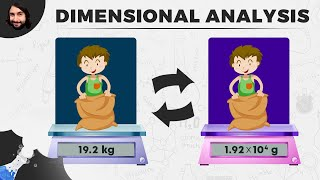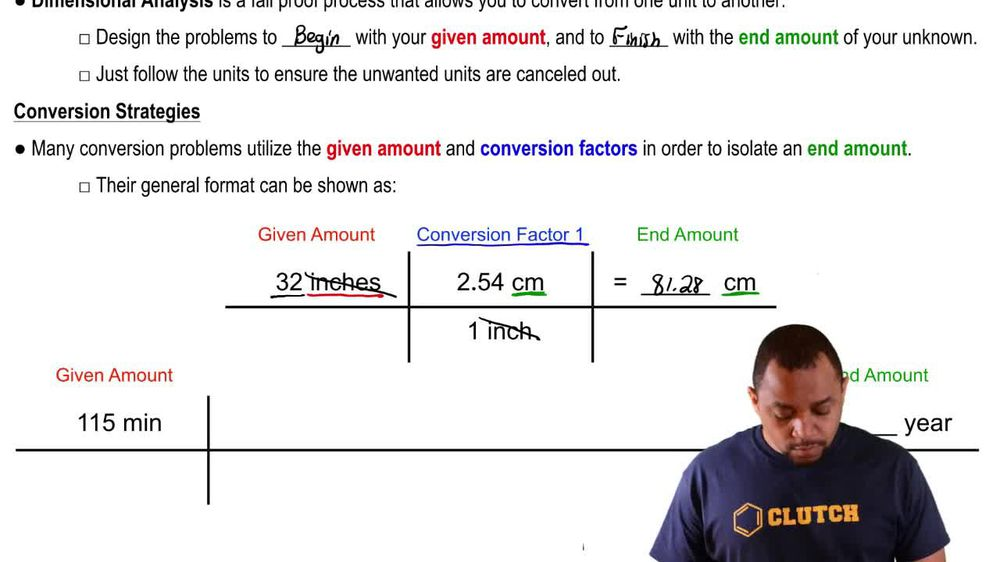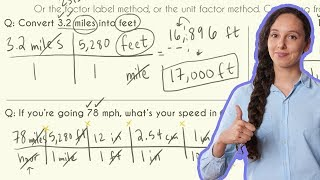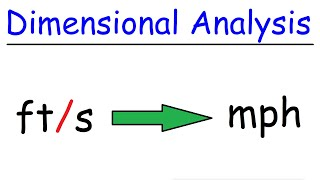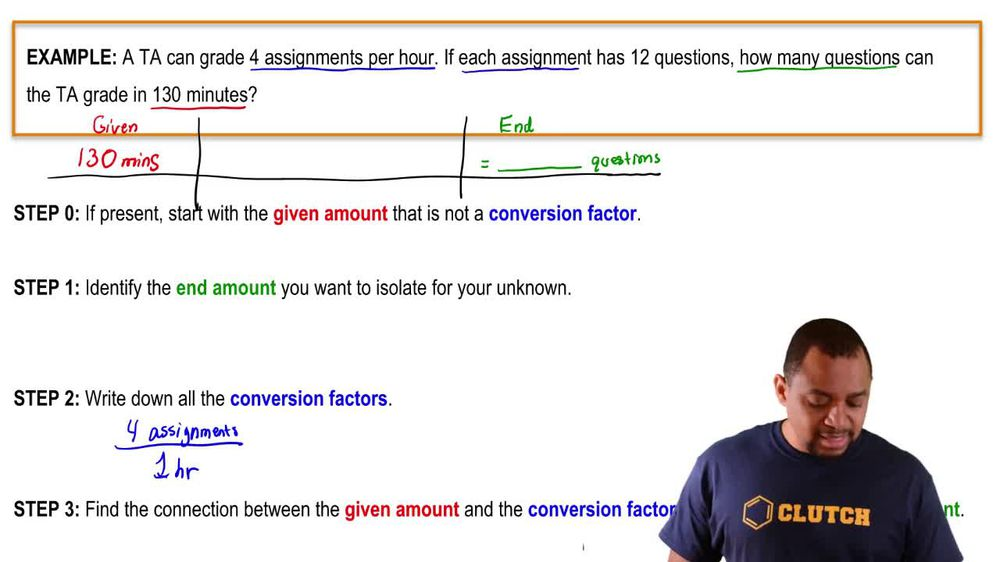Start typing, then use the up and down arrows to select an option from the list.
1. 1. Intro to General Chemistry2. Dimensional Analysis
Problem

# Convert the following quantities into SI units with the correct number of significant figures. (b) 66.31 lb

Relevant Solution2m
Play a video:
the english system uses troy weights to measure precious metals and gems counseling the equivalent of one troy pound in kilograms and in pounds. Alright so they're telling us to start out with a troy pound. If you look at our conversion factors, where do we see troy pound? We see it here in the third conversion factor where it relates troy pounds two troy ounces. So we're gonna stay here one troy pound which I'll abbreviate as Tp And the conversion factor is one troy pound Is equivalent to 12 troy ounces. Which I'll abbreviate as Tokyo. Now I have troy ounces. Where else do I see troy ounces? We see troy ounces connects us to hear the second conversion factor Troyoz. One troy ounce is equal to 20 penny. Wait okay so troy troy pounds cancel out troy ounces left. So now we're gonna say that one troy ounce is equal to penny. Wait. Alright so now that I have penny weights, where else can I go? We see that penny weight is connected to the first conversion factor where one penny weight is equal to 24 grains. Alright so we want penny weights to cancel out so one penny wait, Is equal to 24 grains. Now where do we see grains? We see grains in the fourth conversion factor right over here so one grain is equal to this many grams. So we're gonna say one grain is equal to 0.648 g. And this is important because if we know grams we can convert grams to kilograms. So remember one kg is 10 to the three. So this comes out to a . kilograms. And if we know kilograms, then we know pounds because there's a conversion factor that's not listed here, but connects them together. And so we're gonna do that here, we're going to say .373 kg. Okay. And the conversion factor here is that one kg Is equivalent to 2.2046 lbs. So this comes out .8-2 lbs. Let me my answer for pounds.